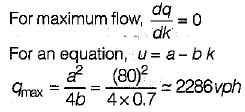Courses

# Traffic Engineering

## 10 Questions MCQ Test Mock Test Series for Civil Engineering (CE) GATE 2020 | Traffic Engineering

Description
This mock test of Traffic Engineering for Civil Engineering (CE) helps you for every Civil Engineering (CE) entrance exam. This contains 10 Multiple Choice Questions for Civil Engineering (CE) Traffic Engineering (mcq) to study with solutions a complete question bank. The solved questions answers in this Traffic Engineering quiz give you a good mix of easy questions and tough questions. Civil Engineering (CE) students definitely take this Traffic Engineering exercise for a better result in the exam. You can find other Traffic Engineering extra questions, long questions & short questions for Civil Engineering (CE) on EduRev as well by searching above.
QUESTION: 1

### Select the correct statement.

Solution:

Traffic capacity is the ability of a road to accommodate traffic volume. It is expressed as the maximum number of vehicle in a lane or a road that can pass a given point in a unit time, usually an hour.

QUESTION: 2

Solution:
QUESTION: 3

### Which of the following shows skewed and staggered form of intersection?

Solution:
QUESTION: 4

The entrance and exit curves of a rotary have

Solution:

Vehicles leaving the rotary would accelerate to the speed of the radiating roads and hence the exit curves should be of larger radii than entry curves. Hence the pavement width at entrance curve will be higher than at exit curve as the radius of the former is less than the latter.

QUESTION: 5

Maximum number of vehicles can be parked with

Solution:

Angle parking or parallel parking may be allowed in the kerb parking. Angle parking may be at angle 30, 60 or 90 degrees. Angle parking accommodates more vehicles per unit length of kerb and maximum vehicles that can be parked is with an angle of 90 degree.

QUESTION: 6

Moving car observer method is a procedure

Solution:
QUESTION: 7

Desire lines are drawn based on

Solution:

Desire lines are plotted which is a graphical representation prepared in almost all O and D surveys, Desire lines are straight lines connecting the origin points with destinations. The width of such desire lines is drawn proportional to the number of trips in both directions.

QUESTION: 8

The PCU (passenger car unit) value for car on an urban road is

Solution:

The PCU may be considered as a measure of the relative space requirement of a vehicle class compared to that of a passenger car under a specified set of roadway, traffic and other conditions. If the addition of one vehicle of a particular class in the traffic stream produces the same effect as that due to the addition of one passenger car, then that vehicle class is considered equivalent to the passenger car with a PCU value equal to 1.0.

QUESTION: 9

Traffic flow equation for a section of road is u = 80 - 0.7 K where ‘u’ is the speed in kmph and ‘K’ is the density in vpkm (vehicles per km). The maximum expected flow is

Solution:

Traffic flow equation:
u = 80 - 0.7k
Since flow, q = u · k = 80 k - 0.7 k2QUESTION: 10

During measurement in spot speed study, a total of 1000 vehicles were observed. 85 per centile and 15 percentile speeds were obtained as 40 kmph and 10 kmph respectively. The number of vehicles moving between speeds of 10 kmph and 40 kmph would be:

Solution:

Percentile speed gives cumulative percentage of vehicle travelling at or below that speed.
Between 85 percentile and 15 percentile speed, 70% of vehicles will be travelling. So 700 vehicles have speed between 10 kmph and 40 kmph.
The difference between 85 percentile speed and 15 percentile speed gives quality of flow.